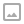## Python - Magic Methods

·   ·  ☕ 4 min read

A complete example

"""
magicmethods.py
Want to try out the examples? Don't want to type them up yourself? Never worry,
magicmethods.py is a convenient Python module with all the class definitions
for the examples in the magic methods guide in it.
"""

# FileObject class, demonstrating __init__ and __del__
from os.path import join

class FileObject:
"""Wrapper for file objects to make sure the file gets closed on deletion."""

def __init__(self, filepath="~", filename="sample.txt"):
# open a file filename in filepath in read and write mode
self.file = open(join(filepath, filename), "r+")

def __del__(self):
self.file.close()
del self.file

# Word class, demonstrating __new__, comparisons
class Word(str):
"""Class for words, defining comparison based on word length."""

def __new__(cls, word):
# Note that we have to use __new__. This is because str is an immutable
# type, so we have to initialize it early (at creation)
if " " in word:
print "Value contains spaces. Truncating to first space."
word = word[:word.index(" ")] # Word is now all chars before first space
return str.__new__(cls, word)

def __gt__(self, other):
return len(self) > len(other)
def __lt__(self, other):
return len(self) < len(other)
def __ge__(self, other):
return len(self) >= len(other)
def __le__(self, other):
return len(self) <= len(other)

# AccessCounter class, demonstrating __setattr__, __getattr__, and __delattr__
class AccessCounter:
"""A class that contains a value and implements an access counter.
The counter increments each time the value is changed."""

def __init__(self, val):
self.__dict__["counter"] = 0
self.__dict__["value"] = val

def __setattr__(self, name, value):
if name == "value":
self.__dict__["counter"] += 1
self.__dict__["value"] = value

def __delattr__(self, name):
if name == "value":
self.__dict__["counter"] += 1
del self.__dict__["value"]

# FunctionalList class, demonstrating __len__, __getitem__, __setitem__, __delitem__,
# __iter__, and __reversed__
class FunctionalList:
"""A class wrapping a list with some extra functional magic, like head,
tail, init, last, drop, and take."""

def __init__(self, values=None):
if values is None:
self.values = []
else:
self.values = values

def __len__(self):
return len(self.values)

def __getitem__(self, key):
# if key is of invalid type or value, the list values will raise the error
return self.values[key]

def __setitem__(self, key, value):
self.values[key] = value

def __delitem__(self, key):
del self.values[key]

def __iter__(self):
return iter(self.values)

def __reversed__(self):
return reversed(self.values)

def append(self, value):
self.values.append(value)
# get the first element
return self.values
def tail(self):
# get all elements after the first
return self.values[1:]
def init(self):
# get elements up to the last
return self.values[:-1]
def last(self):
# get last element
return self.values[-1]
def drop(self, n):
# get all elements except first n
return self.values[n:]
def take(self, n):
# get first n elements
return self.values[:n]

# Entity class demonstrating __call__
class Entity:
"""Class to represent an entity. Callable to update the entity"s position."""

def __init__(self, size, x, y):
self.x, self.y = x, y
self.size = size

def __call__(self, x, y):
"""Change the position of the entity."""
self.x, self.y = x, y

# snip...

# Wrapper class to close an object in a with statement
class Closer:
"""A context manager to automatically close an object with a close method
in a with statement."""

def __init__(self, obj):
self.obj = obj

def __enter__(self):
return self.obj # bound to target

def __exit__(self, exception_type, exception_val, trace):
try:
self.obj.close()
except AttributeError: # obj isn"t closable
print "Not closable."
return True # exception handled successfully

# Classes to represent descriptors and their use
class Meter(object):
"""Descriptor for a meter."""

def __init__(self, value=0.0):
self.value = float(value)
def __get__(self, instance, owner):
return self.value
def __set__(self, instance, value):
self.value = float(value)

class Foot(object):
"""Descriptor for a foot."""

def __get__(self, instance, owner):
return instance.meter * 3.2808
def __set__(self, instance, value):
instance.meter = float(value) / 3.2808

class Distance(object):
"""Class to represent distance holding two descriptors for feet and
meters."""
meter = Meter()
foot = Foot()

# Class to demo fine-tuning pickling
import time

class Slate:
"""Class to store a string and a changelog, and forget its value when
pickled."""

def __init__(self, value):
self.value = value
self.last_change = time.asctime()
self.history = {}

def change(self, new_value):
# Change the value. Commit last value to history
self.history[self.last_change] = self.value
self.value = new_value
self.last_change = time.asctime()

def print_changes(self):
print "Changelog for Slate object:"
for k, v in self.history.items():
print "%s\t %s" % (k, v)

def __getstate__(self):
# Deliberately do not return self.value or self.last_change.
# We want to have a "blank slate" when we unpickle.
return self.history

def __setstate__(self, state):
# Make self.history = state and last_change and value undefined
self.history = state
self.value, self.last_change = None, NoneWRITTEN BY
Ohidur Rahman Bappy
📚Learner 🐍 Developer